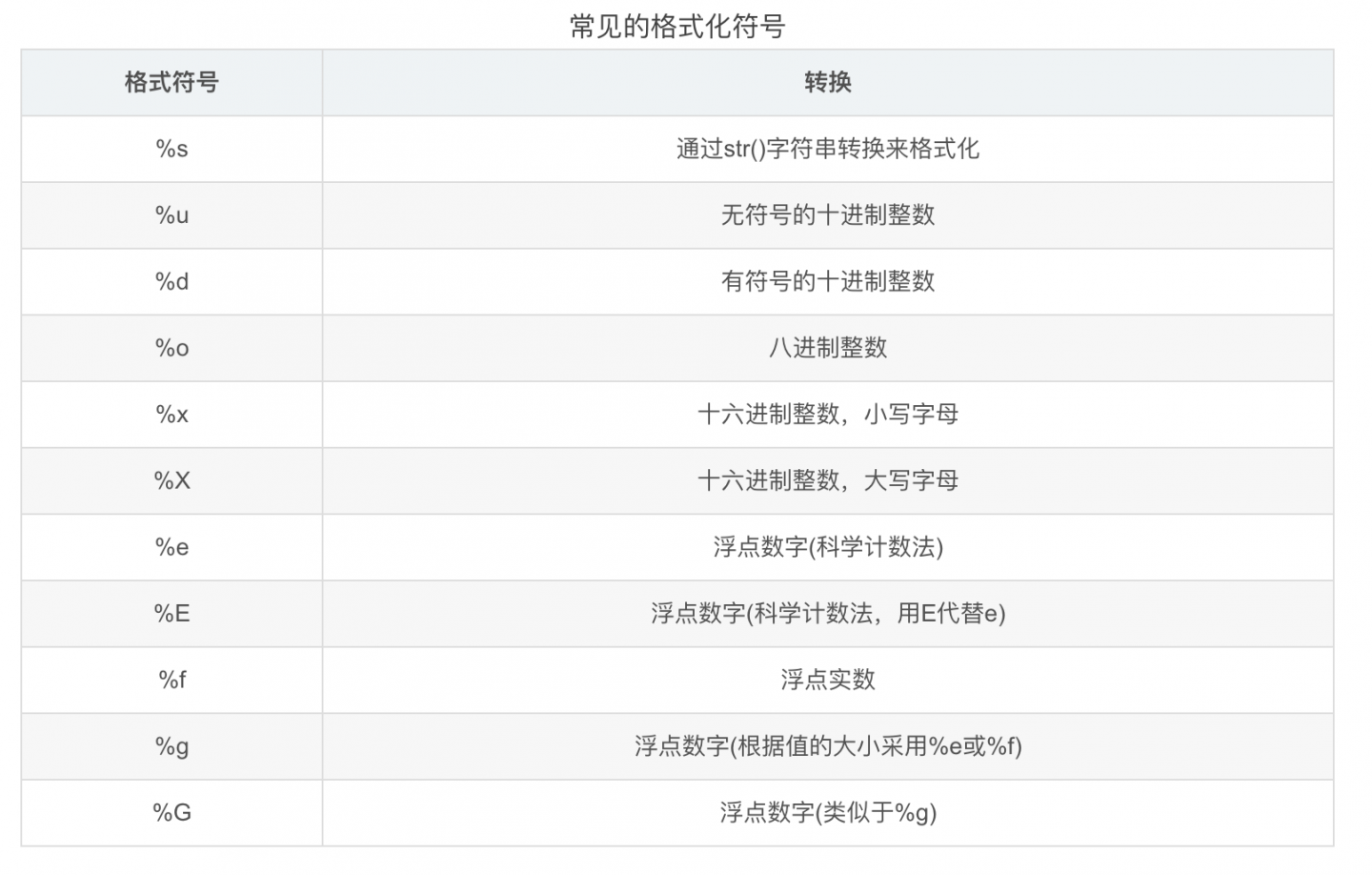# Python 字符串拼接的四种方法

## 一、算术运算符拼接

（1）+算术运算符
+运算符在Python中可以用作数学计算，例如：

num1 = 1
num2 = 2
print(num1 + num2) # 3


s1 = '我是'
s2 = '大帅比'
print(s1 + s2) # 我是大帅比

## 二、format方法

s1 = '小明'
s2 = 18
s3 = 1.75
s4 = True
print('{}今年{}岁了，身高{}米，是个成年的{}男人。'.format(s1, s2, s3, s4))
# 小明今年18岁了，身高1.75米，是个成年的True男人。


s1 = '小明'
s2 = 18
s3 = 1.75
s4 = True
print('{}今年{}岁了，身高{}米，是个成年的男人。'.format(s1, s2))
###
IndexError: Replacement index 2 out of range for positional args tuple


## 三、百分号操作符

Python还可以通过百分号加上一个字母（如%s%d等等），来实现字符串的拼接。

s1 = 'python'
print('人生苦短，我只用%s'%s1)
# 人生苦短，我只用Python


s1 = '张三'
s2 = 24
s3 = 1.8
s4 = True
print('我叫%s,今年%s岁，是个%s米的大帅比，你觉得我说的%s吗？'%(s1, s2, s3, s4))
# 我叫张三,今年24岁，是个1.8米的大帅比，你觉得我说的True吗？## 四、特殊符号f

s1 = '博士'
s2 = 30
print(f'小红是一个{s1}，刚毕业就获得了一个年薪{s2}万的工作。')
# 小红是一个博士，刚毕业就获得了一个年薪30万的工作。


f 和 format 方法有点相似，也是挖坑，但是 f 是现挖现填，相比 format 来说更不容易填错坑。

## 五、总结

Python中实现对字符串拼接的四种方法：
（1）通过 + 或者 * 运算符来实现拼接。
（2）通过 format 方法来进行格式化填空，但是空可以少，值不能少，要确保每个空都会有一个对应的值。
（3）通过百分号来进行字符串格式化拼接，和 format 不一样的是，百分号是有几个空，就填几个值，值不能多，也不能少。
（4）通过特殊符号 f 进行字符串格式化拼接，和百分号方法一样，有几个空就填几个值，空里面不能没有值。

Python字符串拼接的四种方法